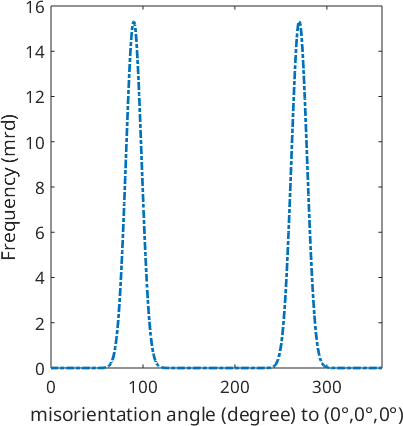Plot Types edit page

## A Sample ODFs

Let us first define a model ODF to be plotted later on.

and simulate some EBSD data

## Scatter Plots

In a scatter plots individual points are plotted. This plot is usually applied when individual orientations or pole figure measurements are visualized.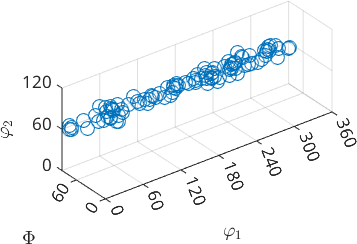Three-dimensional vectors, Miller indices, spherical grids are plotted as single markers in a spherical projection. The shape, size, and color of the markers can be adjusted using the following parameters (see also scattergroup_properties)

Marker, MarkerSize, MarkerFaceColor, MarkerEdgeColor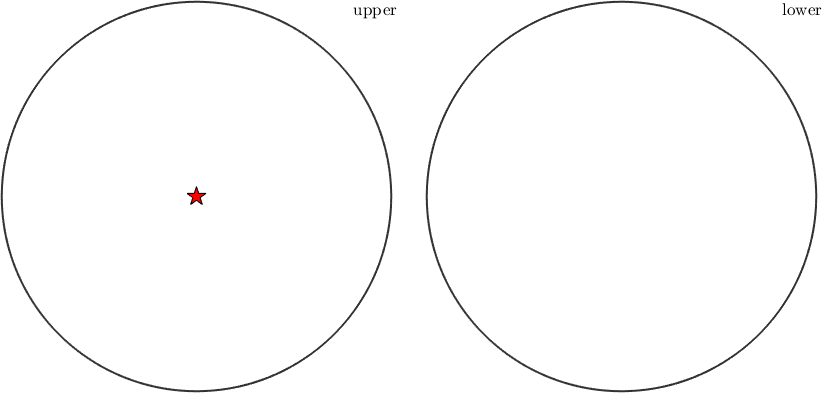One can also assign a label to a marker. The main options controlling the label are (see text_properties)

Label, Color, BackgroundColor, FontSize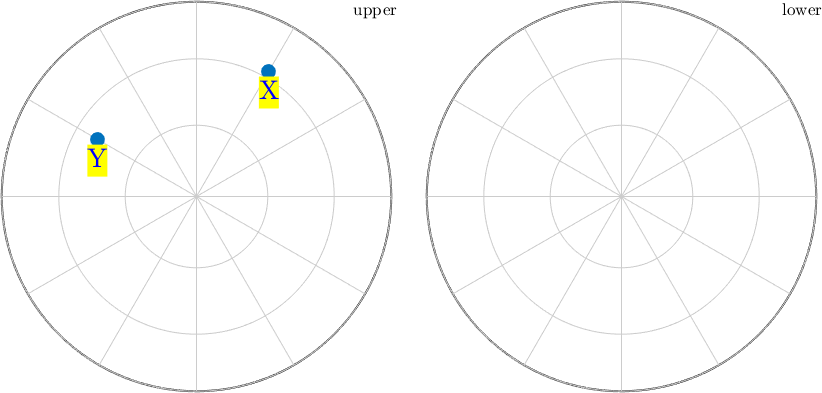A scatter plot is also used to draw raw pole figure data. In this case, each datapoint is represented by a single dot colored accordingly to the intensity.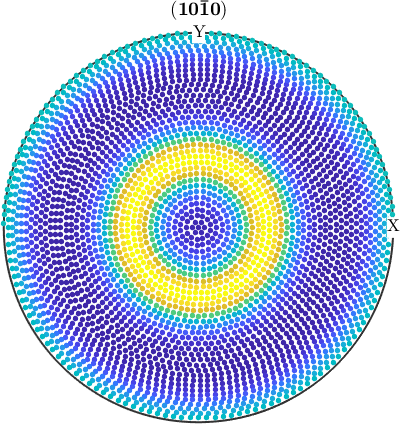## Contour Plots

Contour plots are plots consisting only of contour lines and are mainly used for pole figure and ODF plots. The number or exact location of the contour levels can be specified as an option. See contourgroup properties for more options!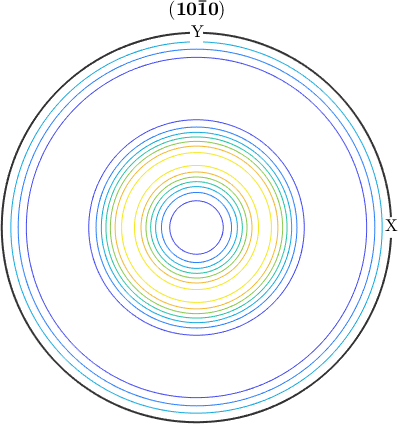## Filled Contour Plots

Filled contour plots are obtained by the option contourf. Again you may pass as an option the number of contour lines or its exact location.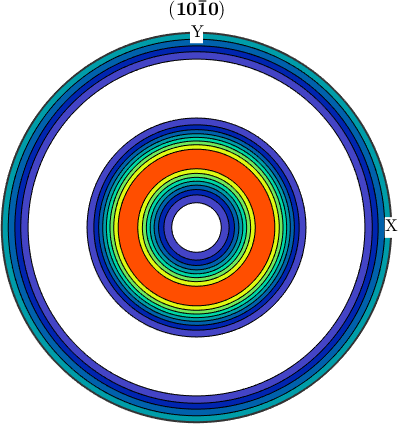## Smooth Interpolated Plots

The default plotting style for pole figures and ODFs is smooth. Which results in a colored plot without contour lines. Here one can specify the resolution of the plot using the option resolution.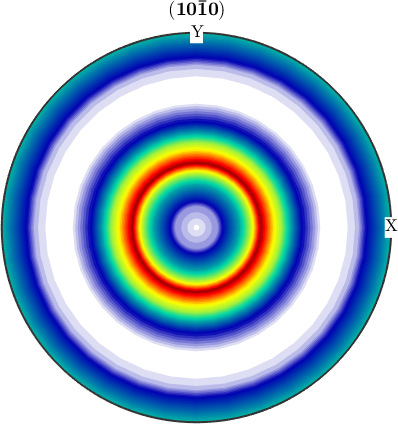## Line Plots

Line plots are used by MTEX for one-dimensional ODF plots, plots of Fourier coefficients and plots of kernel functions. They can be customized by the standard MATLAB linespec options. See linespec!